# Survival Models¶

As the name implies, survival analysis often deals with analyzing mortality event data. More generally, however, it encompasses all manner of failure events, and sometimes includes non-failure events: the contraction of a disease, changes in governments, the occurrence of divorce. It can even be used to model non-failure events, such as the scoring of goals in a sporting event, or the duration of a pregnancy.

As failure events are binary, the simplest approach is to use a binomial model as a likelihood, essentially turning the problem into a logistic regression. This formulation allows for the incorporation of covariates that may be predicitve of the events of interest. As an interesting case study of using binary loigistic regression to predict survival, we will look at the passengers of the RMS Titanic (Eaton & Haas 1995), and estimate the contribution of several variables recorded on the ship's register. This dataset has been used extensively as a test case for predictive modeling, including a Kaggle competition, and is available online in several locations, including the Vanderbilt University Department of Biostatistics website.

In :
import pandas as pd



Some potential predictive variables of interest include sex and age, the passenger class pclass, the number of parents or children of the passenger onboard parch, and the number of siblings or spouses of the passenger sibsp.

In :
%matplotlib inline
import seaborn as sb

titanic['female'] = titanic.sex == 'female'

sb.pairplot(titanic[['age', 'female', 'pclass', 'parch', 'sibsp', 'survived']].dropna(),
hue='survived', size=1)

Out:
<seaborn.axisgrid.PairGrid at 0x10c1dedd8>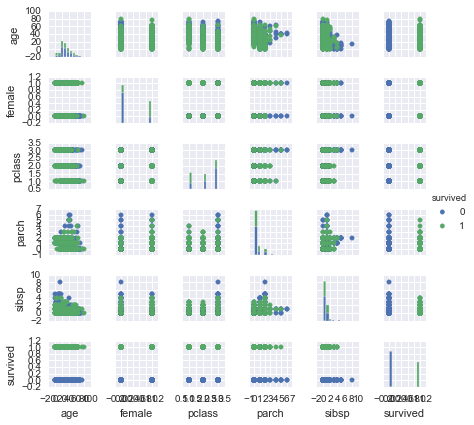It is helpful, prior to fitting a model, to transform some of the variables. Notice above that we recoded the variable sex from a string type to a boolean type, and renamed it female, which makes it obvious what the ones and zeros represent. Looking at the parch and sibsp variables, as one anticipates, the number of related passengers dorps off steeply after one or two, so we will want to aggregate the higher counts. In each case, let's aggregate all counts of two or more.

In :
titanic.parch.value_counts()

Out:
0    1002
1     170
2     113
3       8
5       6
4       6
9       2
6       2
dtype: int64
In :
titanic.sibsp.value_counts()

Out:
0    891
1    319
2     42
4     22
3     20
8      9
5      6
dtype: int64
In :
titanic.loc[titanic.parch>2, 'parch'] = 2
titanic.loc[titanic.sibsp>2, 'sibsp'] = 2


Next, we will standardize the age variable, so that the baseline is interpretable as the average age, and the parameters as change per standard deviation from average.

In :
titanic['ages'] = (titanic.age - titanic.age.mean()) / titanic.age.std()


We will also recode pclass so that they are in reverse order, and 3rd class (the most prevalent) is the baseline.

In :
titanic['passenger_class'] = titanic.pclass.replace({3:0, 2:1, 1:2})


Finally, we may be interested in some interaction effects among the predictors. For example, the effect of passenger class on survival may vary by gender.

In :
titanic['femaleXpclass'] = titanic.female*titanic.passenger_class


We can specify a binary logistic model in PyMC using a Bernoulli likelihood, and modeling the corresponding probability of survival p as a linear function of our set of variables, inverse-logit transformed to place them on the unit interval.

In :
varnames = ['survived', 'passenger_class', 'female', 'ages', 'parch', 'sibsp', 'femaleXpclass']

In :
from pymc import Bernoulli, deterministic, Normal, invlogit
import numpy as np

def titanic_model(dataset):

# Extract variables and drop missing values
X = dataset[varnames].dropna()

# Extract response
survived = X.pop('survived').values

k = X.shape

β = Normal('β', np.zeros(k+1), np.ones(k+1)*0.001, value=np.zeros(k+1))

@deterministic
def p(b=β):
return invlogit(b + b[1:].dot(X.T.values))

y = Bernoulli('y', p, value=survived, observed=True)

return locals()

In :
from pymc import MCMC

M_bin = MCMC(titanic_model(titanic))

In :
M_bin.sample(20000, 10000)

 [-----------------100%-----------------] 20000 of 20000 complete in 19.3 sec
In :
from pymc import Matplot

Matplot.summary_plot(M_bin.β, custom_labels=['intercept'] + varnames[1:])

Could not calculate Gelman-Rubin statistics. Requires multiple chains of equal length.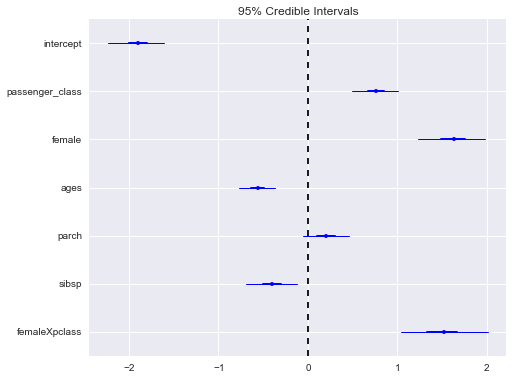The resulting forest plot suggests better expected probability of survival with better passenger class, female gender, and particularly for females in higher passenger clas categories. Whereas, survival declines with age and more siblings or spouses on board. The effect of parents and children is equivocal.

## Non-linear Effects¶

In order to avoid making the assumption that the relationship between age and survival is linear, we will modify the model above to account for this potential non-linearity. There are several possible approaches for this, including splines and Gaussian processes (which we will cover in Chapter 9). We will take a very simple approach and fit a polynomial function for age, which involves a very simple modification to the model, adding two parameters for the quadratic and cubic age terms.

In :
def titanic_poly(dataset):

# Extract variables and drop missing values
X = dataset[varnames].dropna()

# Extract response
survived = X.pop('survived').values

X['age2'] = X.ages**2
X['age3'] = X.ages**3

k = X.shape

β = Normal('β', np.zeros(k+1), np.ones(k+1)*0.001, value=np.zeros(k+1))

@deterministic
def p(b=β):
return invlogit(b + X.dot(b[1:]).values)

y = Bernoulli('y', p, value=survived, observed=True)

return locals()

In :
M_poly = MCMC(titanic_poly(titanic))

In :
M_poly.sample(20000, 10000)

 [-----------------100%-----------------] 20000 of 20000 complete in 20.4 sec
In :
Matplot.summary_plot(M_poly.β, custom_labels=['intercept'] + M_poly.X.columns.tolist())

Could not calculate Gelman-Rubin statistics. Requires multiple chains of equal length.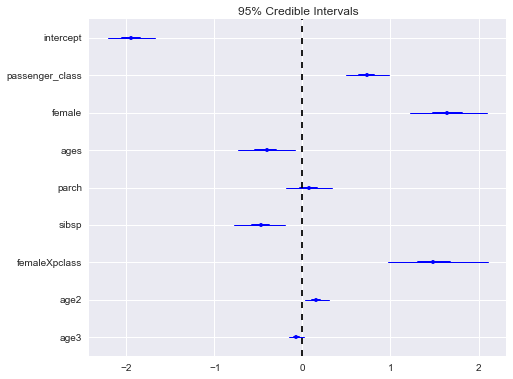The resulting fit suggests a slight curvilinear relationship with age.

In :
import pylab as pl

xvals = np.linspace(titanic.ages.min(), titanic.ages.max())

beta_age = M_poly.β.stats()['mean'][[3,7,8]]
pl.plot(xvals, beta_age.dot([xvals, xvals**2, xvals**3]))
pl.xlabel('Standardized Age'); pl.ylabel('Age effect');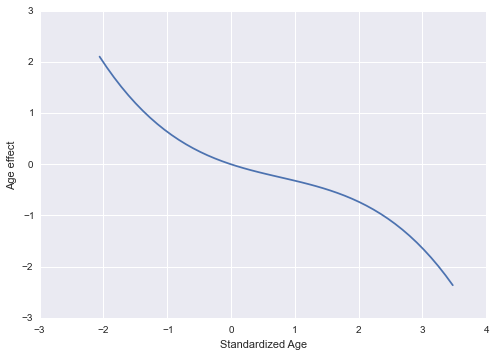## Imputation of missing values¶

The biggest shortcoming of these models is not the assumptions regarding the functional form, but rather, how missing data have been treated. In the Titanic dataset, there were several passengers that did not have their ages recorded. The simplest solution is to discard these records from the analysis, which we did (notice the dropna() method called when the variables were extracted). However, this so-called complete-case analysis is not optimal; it discards the partial information contained on those records, and may lead to bias, depending on the nature of the missingness.

Instead, we can impute these missing values by treating them as unknown quantities, just as we would with a variable. All we need is a model for age, and to include this as part of the overall model. In a Bayesian modeling framework, missing data are accommodated simply by treating them as unknown model parameters. Values for the missing data $\tilde{y}$ are estimated naturally, using the posterior predictive distribution:

$$p(\tilde{y} | y) = \int p(\tilde{y}|\theta) p(\theta | y) d\theta$$

This describes additional data $\tilde{y}$, which may either be considered unobserved data or potential future observations. We can use the posterior predictive distribution to model the likely values of missing data.

Here is the distribution of known ages. Although this distribution looks slighlty bimodal, for simplicity we will use a truncated normal distribution to model age, and use this to impute the missing values. Note that this implicitly assumes a missing completely at random (MCAR) scenario, which may or may not be true here.

In :
titanic.ages.dropna().hist(bins=40)

Out:
<matplotlib.axes._subplots.AxesSubplot at 0x1462c8438>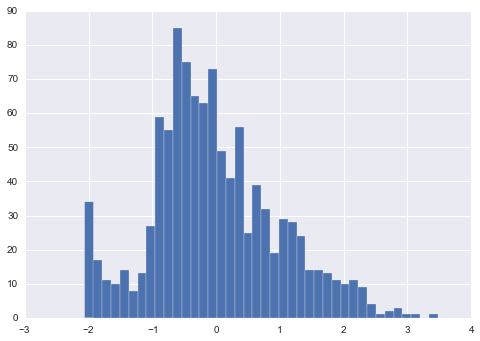PyMC supports imputation via masked arrays in Numpy. A Numpy masked_array simply combines a standard array with a binary mask that hides invalid (here, missing) values. Specifically, when an element of the mask is True, the corresponding element of the array is said to be masked (invalid).

In :
from numpy.ma import masked_values

example_data = [3, 5, -999, 17, -3]

Out:
masked_array(data = [3 5 -- 17 -3],
mask = [False False  True False False],
fill_value = -999)

Here, the value -999 is a sentinel value that represents elements that are missing.

A PyMC data stochastic node that is passed a masked_array as treats the unmasked values as regular data, but then turns the masked values into Stochastic nodes, to be estimated with the rest of the model. Thus, the node age below is a hybrid that includes both unobserved and observed stochastic elements.

The missing values in the Pandas DataFrame are represented by nan values. We will replace these with an arbitrary sentinel value that is a valid standardized age. This is necessary because the chosen value will act as the initial value for the unobserved parts of the stochastic node, so leaving the missing value as nan may raise an error when the model is initialized. Here, we use zero as the sentinel value because there are no values that are exactly zero in the non-missing data (recall that the ages were standardized).

In :
from pymc import TruncatedNormal

def titanic_imp(dataset):

# Extract variables and drop missing values
X = dataset[varnames]

# Extract response
survived = X.pop('survived').values

# Imputation of age
# Negative binomial parameters
mu = Normal('mu', 0, 0.01, value=0)
tau = Exponential('tau', 1, value=1)
# Imputation distribution
age = TruncatedNormal('age', mu=mu, tau=tau, a=-2.1, b=10000, value=age_masked, observed=True)

k = X.shape + 3

β = Normal('β', np.zeros(k+1), np.ones(k+1)*0.001, value=np.zeros(k+1))

@deterministic
def p(b=β, age=age):
A = [age, age**2, age**3]
return invlogit(b + X.dot(b[1:-3]).values + np.dot(b[-3:], A))

y = Bernoulli('y', p, value=survived, observed=True)

return locals()

In :
M_titanic_imp = MCMC(titanic_imp(titanic))

In :
M_titanic_imp.sample(20000, 10000)

 [-----------------100%-----------------] 20000 of 20000 complete in 43.2 sec
In :
Matplot.summary_plot(M_titanic_imp.β, custom_labels=['intercept'] + M_poly.X.columns.tolist())

Could not calculate Gelman-Rubin statistics. Requires multiple chains of equal length.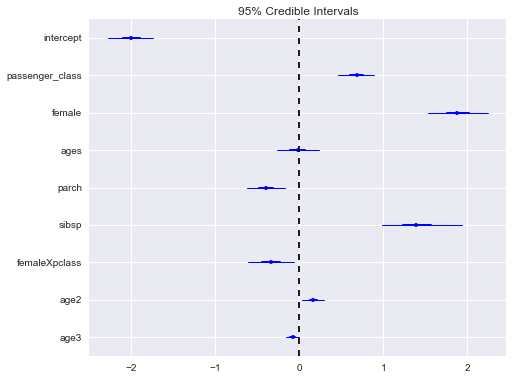Below is the posterior distribution of one of the missing ages:

In :
pl.hist(M_titanic_imp.age.trace(), bins=80);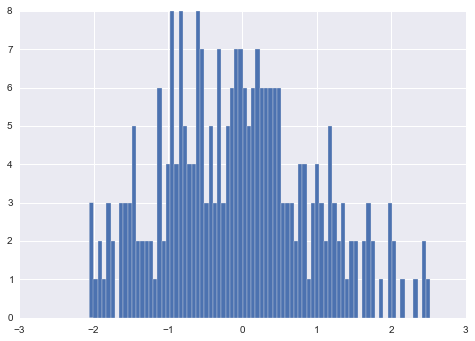## Parametric survival models¶

In the Titanic example, we were able to use a simple binomial model for the survival rate because the disaster was a discrete event. That is, the outcomes of each passenger on the passenger list was known shortly after the event occurred. In many biomedical situations, however, the study endpoint might not be reached for all subjects by the time the study is completed. The fate of these individuals, which we refer to as right censored is unknown to us, but treating them as known will bias estimates of the survival rate and of any treatment we may be studying.

When we are dealing with censored events, survival analyses typically shift to analyzing time to events instead of the occurrence or non-occurence of events.

### Some definitions¶

Density function describes the distribution of times to events:

$$f(t) = Pr(T=t)$$

(or continuous equivalent)

Survival function describes the probability of surviving to time $t$ or beyond:

$$S(t) = Pr(T \ge t)$$

Hazard function describes the instantaneous failure rate, that is, the probability of an event at time $t$ given that it has not happend prior to $t$:

$$\lambda(t) = \frac{f(t)}{S(t)}$$

Cumulative hazard function integrates the hazard from time zero until $t$:

$$\Lambda(t) = \int_0^t \lambda(u) du$$

### Exponential model¶

A simple parametric approach to modeling failure times is the exponential model. This model assumes that failure times are distributed identically and independently as exponential random variables:

$$f(t_i|\lambda) = \lambda \exp(-\lambda t_i)$$

by definition, this implies that the survival function is:

$$S(t_i|\lambda) = \exp(-\lambda t_i)$$

Accounting for censoring, from this we can write the likelihood function for $\lambda$ as:

$$L(\lambda|\mathbf{t},\mathbf{\nu}) = \prod_{i=1}^n f(t_i|\lambda)^{\nu_i} S(t_i|\lambda)^{1-\nu_i} = \lambda^{\sum_i \nu_i} \exp\left[-\lambda \sum_i t_i\right]$$

We typically wish to model $\lambda$ as a function of one or more covariates, such as a treatment.

$$\lambda_i = \phi(x_i^{\prime} \mathbf{\beta})$$

Substituting and re-arranging gives:

$$L(\lambda|\mathbf{t},\mathbf{\nu}, \mathbf{\beta}) = \exp\left[\sum_i \nu_i x_i^{\prime} \mathbf{\beta}\right] \exp\left[-\sum_i t_i \exp(x_i^{\prime} \mathbf{\beta})\right]$$

Note, however that the hazard is constant (not time-varying), which is the important implication of the exponential model.

Below is an exponential model fit to data from the E1684 melanoma clinical trial comparing the effect of high-dose interferon to a control arm (Kirkwood et al. 2000).

In :
%run -i data/melanoma_data.py

In :
from pymc import observed

def exp_survival_model():

# Convert censoring indicators to indicators for failure event
failure = (censored==0).astype(int)

# Intercept for survival rate
beta0 = Normal('beta0', mu=0.0, tau=0.0001, value=0.0)
# Treatment effect
beta1 = Normal('beta1', mu=0.0, tau=0.0001, value=0.0)

# Survival rates
lam = Lambda('lam', lambda b0=beta0, b1=beta1, t=treat: np.exp(b0 + b1*t))

@observed
def survival(value=t, lam=lam, f=failure):
"""Exponential survival likelihood, accounting for censoring"""
return sum(f*np.log(lam) - lam*value)

return locals()

In :
E = MCMC(exp_survival_model())
E.sample(10000, 5000)

 [-----------------100%-----------------] 10000 of 10000 complete in 1.5 sec
In :
E.beta1.summary()

beta1:

Mean             SD               MC Error        95% HPD interval
------------------------------------------------------------------
-0.261           0.162            0.01             [-0.593  0.047]

Posterior quantiles:

2.5             25              50              75             97.5
|---------------|===============|===============|---------------|
-0.568           -0.37           -0.267         -0.15         0.081


In :
Matplot.plot(E.beta1)

Plotting beta1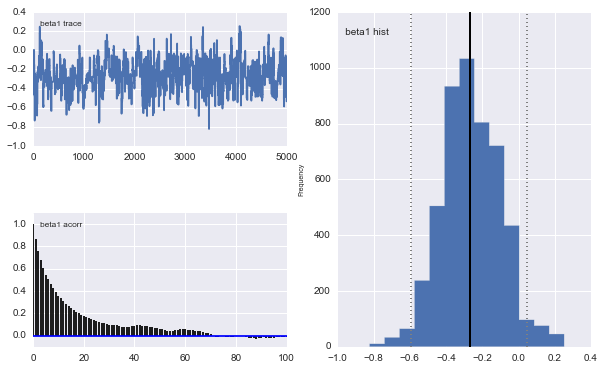### Weibull model¶

If a constant hazard rate is not a reasonable assumption, we can generalize the survivial model to allow the hazard to vary over time. The simplest non-constant model is to allow the hazard to vary linearly with log-time; this is the characteristic of the Weibull survival function.

Dellaportas and Smith (1993) analysed data on photocarcinogenicity in four groups (irradiated control, vehicle control, test substance, positive control), each containing 20 mice, who have recorded a survival time and whether they died or were censored at that time.

In :
# treatment group assignments
group = (0, 0, 0, 0, 0, 0, 0, 0, 0, 0, 0, 0, 0, 0, 0, 0, 0, 0, 0, 0,
1, 1, 1, 1, 1, 1, 1, 1, 1, 1, 1, 1, 1, 1, 1, 1, 1, 1, 1, 1, 2,
2, 2, 2, 2, 2, 2, 2, 2, 2, 2, 2, 2, 2, 2, 2, 2, 2, 2, 2, 3, 3,
3, 3, 3, 3, 3, 3, 3, 3, 3, 3, 3, 3, 3, 3, 3, 3, 3, 3)

# censoring
is_censored = (0, 0, 0, 0, 0, 0, 0, 0, 0, 0, 0, 0, 0, 0, 0, 0, 0, 1, 0, 0,
0, 0, 0, 0, 0, 1, 1, 0, 0, 0, 1, 0, 1, 1, 1, 1, 0, 0, 0, 0, 0,
0, 1, 0, 0, 0, 0, 0, 0, 0, 0, 0, 1, 0, 1, 1, 0, 0, 0, 0, 0, 0,
0, 0, 0, 0, 0, 1, 0, 0, 0, 0, 1, 1, 0, 0, 0, 0, 0, 0)

# censoring time
last_t = (40, 40, 40, 40, 40, 40, 40, 40, 40, 40, 40, 40, 40, 40, 40,
40, 40, 40, 40, 40, 40, 40, 40, 40, 40, 40, 40, 40, 40, 40, 40,
40, 40, 40, 40, 40, 40, 40, 40, 40, 40, 40, 10, 40, 40, 40, 40,
40, 40, 40, 40, 40, 24, 40, 40, 40, 40, 40, 40, 40, 40, 40, 40,
40, 40, 40, 40, 20, 40, 40, 40, 40, 29, 10, 40, 40, 40, 40, 40,
40)

# number of treatment groups
M = 4

# number of individuals
N = 80

# failure time for each mouse
t = (12, 17, 21, 25, 11, 26, 27, 30, 13, 12, 21, 20, 23, 25, 23,
29, 35, 50, 31, 36, 32, 27, 23, 12, 18, 50, 50, 38, 29, 30, 50,
32, 50, 50, 50, 50, 25, 30, 37, 27, 22, 26, 50, 28, 19, 15, 12,
35, 35, 10, 22, 18, 50, 12, 50, 50, 31, 24, 37, 29, 27, 18, 22,
13, 18, 29, 28, 50, 16, 22, 26, 19, 50, 50, 17, 28, 26, 12, 17,
26)


We will use a Weibull distribution to model the survival times:

$$f(x \mid \alpha, \beta) = \frac{\alpha x^{\alpha - 1}\exp(-(\frac{x}{\beta})^{\alpha})}{\beta^\alpha}$$

The baseline hazard function for this model is:

$$\lambda_0(t) = \alpha t^{\alpha-1}$$

if we set $\beta_i = \exp(-\beta^{\prime}z_i)$ then we have:

$$t_i \sim \text{Weibull}(\alpha, \beta_i)$$

In this model, we account for censored observations by using a truncated Weibull, with the censoring time as the lower bound. PyMC does not have a truncated Weibull built-in, but we can easily create one by modfying the model's log-probability with a factor potential. Factor potentials don’t correspond to probabilities of variables conditional on parents, but are simply arbitrary probability terms that can be added to existing models. In our case, we want to constrain the latent (censored) failure times to be later than the last obervation period: $I(t>T)$. Lauritzen et al. (1990) and Jordan (2004) call these factor potentials. Formally, there is no accomodation in Bayesian hierarchical notation for these potentials. They are often used in cases where there is no natural dependence hierarchy.

In PyMC, we can most easily specify a factor potential by adding a @potential decorator to a function that returns a log-probability, given the values of their parents. Potentials have no methods, nor do they have value or trace attributes, because they are not variables. Because of this, they cannot serve as parents of variables, and hence have no children attribute.

In :
from pymc import Weibull, potential

def mice_model():

beta = Normal("beta", 0, 0.0001, value=np.ones(M)*-10)
alpha = Gamma("alpha", 1.0, 0.0001, value=4)

theta = Lambda("theta", lambda b=beta: 1./np.exp(b[np.array(group)]))
median_survival = Lambda("median_survival", lambda b=beta, a=alpha: (np.log(2)*np.exp(-b))**(1/a))
veh_control = Lambda("veh_control", lambda b=beta: b-b)
test_sub = Lambda("test_sub", lambda b=beta: b-b)
pos_control = Lambda("pos_control", lambda b=beta: b-b)

time = Weibull("time", alpha, theta, value=t_masked, observed=True)

@potential
def censor(time=time):
return -np.inf
return 0

return locals()

In :
MM = MCMC(mice_model())

In :
MM.sample(20000, 10000)

 [-----------------100%-----------------] 20000 of 20000 complete in 4.8 sec
In :
Matplot.summary_plot(MM.median_survival)

Could not calculate Gelman-Rubin statistics. Requires multiple chains of equal length.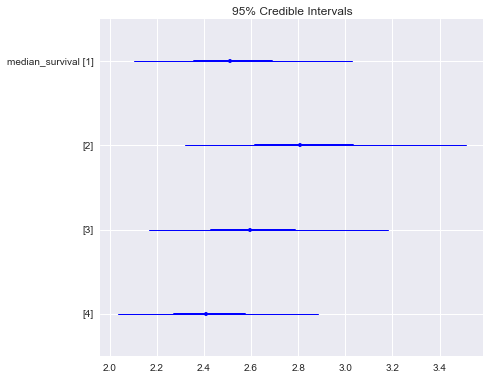## Semiparametric survival models¶

Parametric models are effective if the underlying survival time distribution is known. However, the exact form of the underlying survival distribution is usually unknown and we may not be able to find an appropriate model. Semiparametric survival models relax the assumption of a known, parametric form for the survival function. The most popular semiparametric model is the Cox proportional hazards model, which replaces the assumption of a parametric survival time distribution with an assumption of proportional hazards. In other words, the ratio in the risk of death/failure between two groups does not vary over time.

The proportional hazards assumption implies that the hazard function given a set of covariates $Z$ can be written as a function of a baseline hazard function and a function of only the covariates $g(Z)$. Using a simplified example, where $Z = 1$ for treated and $Z = 0$ for control individuals, and $\lambda_1(t)$ is the hazard rate for the treated group and $\lambda_0(t)$ the hazard for control, then we can write:

$$\lambda_1(t) = \lambda(t|Z=1) = \lambda_0(t)\exp(\beta Z) = \lambda_0(t)\exp(\beta)$$

The Cox model can be re-expressed as a linear model for the log of the hazard ratio:

$$\log\left[\frac{\lambda_i(t)}{\lambda_0(t)}\right] = Z \mathbf{\beta}$$

So, while the baseline hazard is non-parametric, the linear model is parametric, making the overall model semiparametric.

In frequentist applications, the Cox model is estimated using the partial likelihood. In Bayesian applications, since we must always specify a parametric posterior distribution, we must re-express the Cox model in order to estimate it.

### Cox regression in PyMC¶

This example implements the Cox model using the counting process notation introduced by Andersen and Gill (1982)

If $N_i(t)$ is the count of events that have occurred up until time $t$, then we can consider an intensity process that characterizes the incremental increase in $N_i(t)$ over some small interval $dt$:

$$I_i(t) dt = E(dN_i(t) \,| \, N_i(t), Y_i(t), z_i)$$

if subject $i$ fails during $[t,t+dt)$, then $dN_i(t)=1$, making $I_i(t) dt$ a failure rate for this interval. As $dt \rightarrow 0$, this probability converges to the hazard. Here is the proportional hazards form:

$$I_i(t) = Y_i(t) \lambda_0(t) \exp(\beta^{\prime}z_i)$$

Since we cannot estimate $\lambda_0(t)$ non-parametrically, we instead model the increments $dN_i(t)=1$ as a counting process with a Poisson distribution:

$$dN_i(t) \sim Poisson(I_i(t) dt)$$

where the Poisson mean is:

$$I_i(t) dt = Y_i(t) \lambda_0(t) \exp(\beta^{\prime}z_i) d\Lambda_0(t)$$

One approach places a prior on the unknown hazard function $d\Lambda_0(t)=\lambda_0(t)$, but it is cleaner to invoke the "multinomial-Poisson trick", which assumes independent increments in the cumulative hazard function, and whose logarithms are given flat (constant) priors.

$$\log(\lambda_{ij}) = \lambda_i + \beta_j^{\prime} z_i$$

Survival time, in weeks:

In :
obs_t = (1, 1, 2, 2, 3, 4, 4, 5, 5, 8, 8, 8, 8, 11, 11, 12, 12, 15, 17, 22, 23, 6,
6, 6, 6, 7, 9, 10, 10, 11, 13, 16, 17, 19, 20, 22, 23, 25, 32, 32, 34, 35)


Vector of indicator variables for failure (1) or censored (0):

In :
fail = (1, 1, 1, 1, 1, 1, 1, 1, 1, 1, 1, 1, 1, 1, 1, 1, 1, 1, 1, 1, 1, 1, 1, 1, 0, 1,
0, 1, 0, 0, 1, 1, 0, 0, 0, 1, 1, 0, 0, 0, 0, 0)


Vector of indicator variables for treatment (0.5) or placebo (-0.5):

In :
Z = (0.5, 0.5, 0.5, 0.5, 0.5, 0.5, 0.5, 0.5, 0.5, 0.5, 0.5, 0.5, 0.5, 0.5, 0.5, 0.5,
0.5, 0.5, 0.5, 0.5, 0.5, -0.5, -0.5, -0.5, -0.5, -0.5, -0.5, -0.5, -0.5, -0.5, -0.5,
-0.5, -0.5, -0.5, -0.5, -0.5, -0.5, -0.5, -0.5, -0.5, -0.5, -0.5)


Unique failure times, number of observations, and study duration:

In :
t = 1, 2, 3, 4, 5, 6, 7, 8, 10, 11, 12, 13, 15, 16, 17, 22, 23, 35
N_obs = len(obs_t)
T = len(t) - 1


Calculate risk set

In :
Y = np.array([[int(obs >= time) for time in t] for obs in obs_t])


Counting process. Jump = 1 if $\text{obs}_t \in [ t_j, t_{j+1} )$

In :
dN = np.array([[Y[i,j]*(t[j+1] >= obs_t[i])*fail[i] for i in range(N_obs)] for j in range(T)])


Model using Poisson trick:

In :
from pymc import Poisson

def cox_model():

beta0 = Normal('beta0', 0, 0.001, value=np.zeros(T))
beta1 = Normal('beta1', 0, 0.00001, value=0)

def Idt(b0=beta0, b1=beta1):
# Poisson trick: independent log-normal hazard increments
return [[Y[i,j]*np.exp(b0[j] + b1*Z[i]) for i in range(N_obs)] for j in range(T)])

dN_like = Poisson('dN_like', Idt, value=dN, observed=True)

return locals()


Initialize MCMC sampler.

In :
M_cox = MCMC(cox_model())

In :
M_cox.sample(20000, 10000)

 [-----------------100%-----------------] 20000 of 20000 complete in 141.8 sec
In :
M_cox.beta1.summary()

beta1:

Mean             SD               MC Error        95% HPD interval
------------------------------------------------------------------
1.428            0.305            0.011            [ 0.792  1.976]

Posterior quantiles:

2.5             25              50              75             97.5
|---------------|===============|===============|---------------|
0.836            1.22            1.428          1.636         2.041


In :
Matplot.plot(M_cox.beta1)

Plotting beta1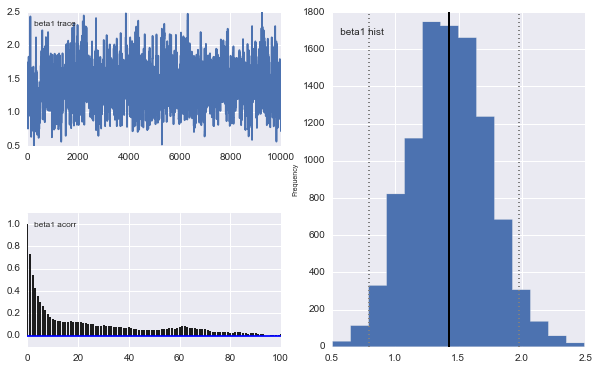Dellaportas P and AFM Smith. Bayesian Inference for Generalized Linear and Proportional Hazards Models via Gibbs Sampling. Applied Statistics. 1993;42(3):443. doi:10.2307/2986324.

Eaton, JP and CA Haas. Titanic: Triumph and Tragedy (2nd Edition). WW Norton, New York, New York. 1995.

Jordan, MI. Graphical models. Statist. Sci. 2004;19(1):140–155.

Kirkwood, JM, JG Ibrahim, VK Sondak, J Richards, LE Flaherty, MS Ernstoff, TJ Smith, U Rao, M Steele, and RH Blum. 2000. High- and low-dose interferon alfa-2b in high-risk melanoma: first analysis of intergroup trial E1690/S9111/C9190. J Clin Oncol. 2000;18(12):2444-2458.

Lauritzen SL, AP Dawid, BN Larsen and H-G Leimer. Independence properties of directed Markov fields. Networks. 1990;20(5):491-505.

In :
from IPython.core.display import HTML
def css_styling():